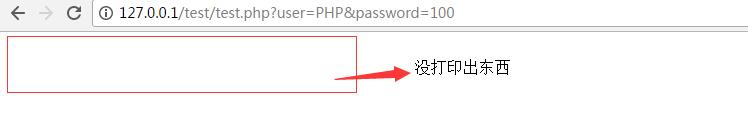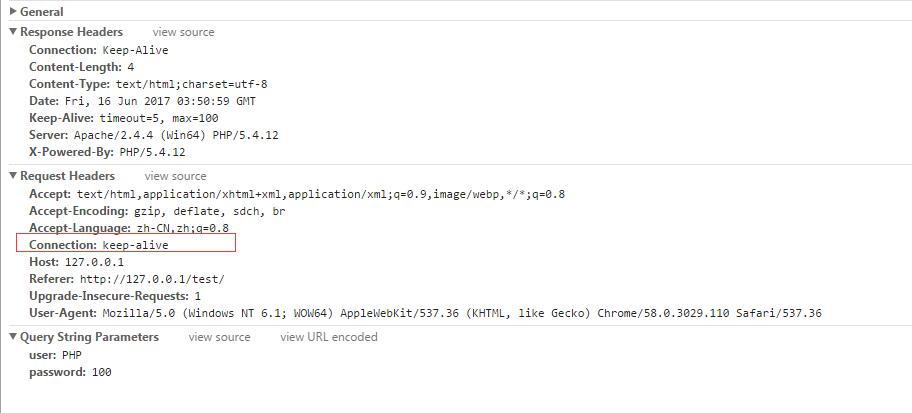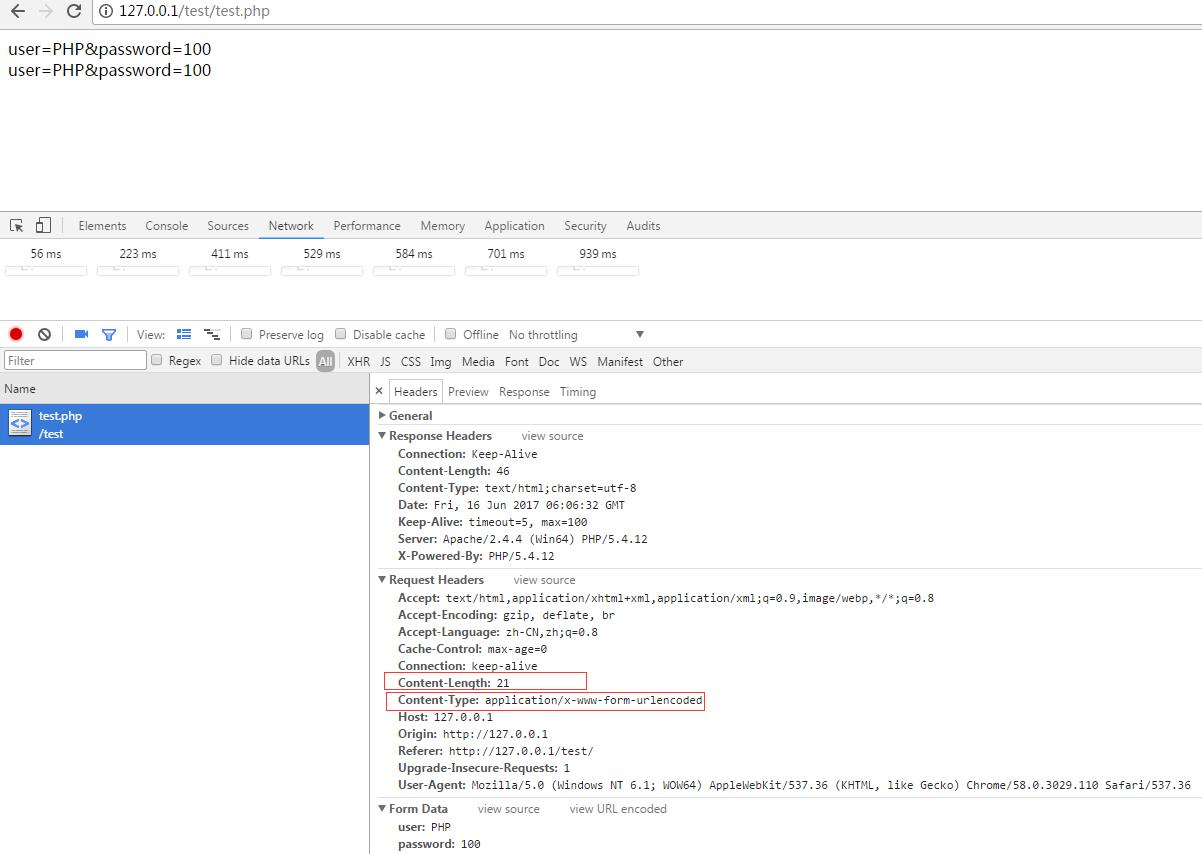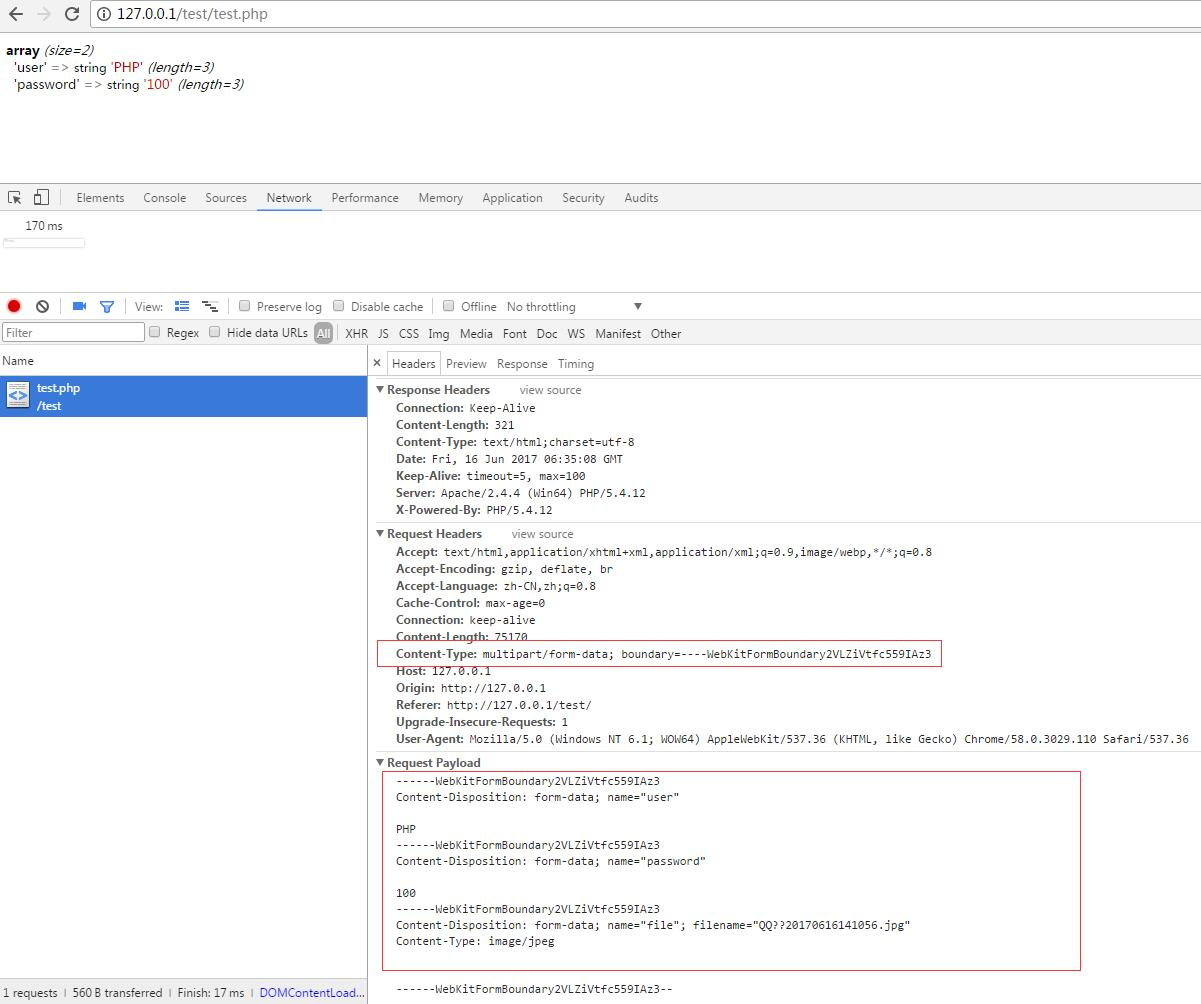# 谈一谈php://input和php://output

php://input 是个可以访问请求的原始数据的只读流。 POST 请求的情况下，最好使用 php://input 来代替 $HTTP_RAW_POST_DATA（原生的post数据），因为它不依赖于特定的 php.ini 指令,内存消耗更少 “php://input allows you to read raw POST data. It is a less memory intensive alternative to$HTTP_RAW_POST_DATA and does not need any special php.ini directives. php://input is not available with enctype=”multipart/form-data”.

“php://input可以读取没有处理过的POST数据。相较于$HTTP_RAW_POST_DATA而言，它给内存带来的压力较小，并且不需要特殊的php.ini设置。php://input不能用于enctype=multipart/form-data” 我们应该怎么去理解这段概述呢?!我把它划分为三部分，逐步去理解。 读取POST数据 不能用于multipart/form-data类型 php://input VS$HTTP_RAW_POST_DATA

PHPer们一定很熟悉$_POST这个内置变量。$_POST与 php://input存在哪些关联与区别呢?另外，客户端向服务端交互数据，最常用的方法除了POST之外，还有GET。既然php://input作 为PHP输入流，它能读取GET数据吗？

GET提交的表单:

index.php

<form action="test.php" method="get">
<input type="text" name="user">
<input type="password" name="password">
<input type="submit">
</form>

test.php

<?php
header("Content-Type:text/html;charset=utf-8");
$file_in = file_get_contents("php://input"); echo$file_in;
echo "<br>";
echo urldecode($file_in); 结果：POST提交表单: index.php <form action="test.php" method="post"> <input type="text" name="user"> <input type="password" name="password"> <input type="submit"> </form> test.php <?php header("Content-Type:text/html;charset=utf-8");$file_in = file_get_contents("php://input");
echo $file_in; echo "<br>"; echo urldecode($file_in);1、GET提交时，不会指定Content-Type和Content-Length，它表示http请求body中的数据是使用http的post方法提交的表单数据，并且进行了urlencode()处理。

POST提交时，Content- Type取值为application/x-www-form-urlencoded时，也指明了Content-Length的值，php会将http请求body相应数据会填入到数 组$_POST，填入到$_POST数组中的数据是进行urldecode()解析的结果。

2，php://input数据，只要Content-Type不为 multipart/form-data(该条件限制稍后会介绍)。那么php://input数据与http entity body部分数据是一致的。该部分相一致的数据的长度由Content-Length指定。
3，仅当Content-Type为application/x-www-form-urlencoded且提交方法是POST方法时，$_POST数据与php://input数据才是”一致”（打上引号，表示它们格式不一致，内容一致）的。其它情况，它们都不一致。 4，php://input读取不到$_GET数据。是因为$_GET数据作为query_path写在http请求头部(header)的PATH字段，而不是写在http请求的body部分。 加了enctype=multipart/form-data的上传文件的时候 index.php <form enctype="multipart/form-data" action="test.php" method="post"> <input type="text" name="user"> <input type="password" name="password"> <input type="file" name="file" id="" /> <input type="submit"> </form> test.php <?php header("Content-Type:text/html;charset=utf-8"); var_dump($_POST);
$file_in = file_get_contents("php://input"); echo$file_in;
echo "<br>";
echo urldecode($file_in); ?> 结果:说明: 从响应输出来比对，$_POST数据跟请求提交数据相符，即$_POST = array (size=2) 'user' => string 'PHP' (length=3) 'password' => string '100' (length=3)。这也跟http请求body中的数据相呼应，同时说明PHP把相应的数据填入$_POST全局变量。而php://input 输出为空，没有输出任何东西，尽管http请求数据包中body不为空。这表示，当Content-Type为multipart/form-data的 时候，即便http请求body中存在数据，php://input也为空，PHP此时，不会把数据填入php://input流。所以，可以确定: php://input不能用于读取enctype=multipart/form-data数据。

test.php

<?php
$raw_post_data = file_get_contents('php://input', 'r');$rtn = ($raw_post_data ==$HTTP_RAW_POST_DATA) ? 1 : 0;
echo $rtn; ?> 结果:说明: 得出的结果输出都是一样的，即都为1，表示php://input和$HTTP_RAW_POST_DATA是相同的。至于对内存的压力，我们这里就不做细致地测试了。有兴趣的，可以通过xhprof进行测试和观察。

1, php://input 可以读取http entity body中指定长度的值,由Content-Length指定长度,不管是POST方式或者GET方法提交过来的数据。但是，一般GET方法提交数据 时，http request entity body部分都为空。
2,php://input 与$HTTP_RAW_POST_DATA读取的数据是一样的，都只读取Content-Type不为multipart/form-data的数据。 php://output is a write-only stream that allows you to write to the output buffer mechanism in the same way as print and echo.(摘自PHP官网) 大概意思:php://output是一个只写流，允许您以与var_dump和echo相同的方式写入输出缓冲区机制。 读取数据输出到浏览器(PHPExcel里的运用) <?php$dir=dirname(__FILE__);//查找当前脚本所在路径

require $dir."/db.php";//引入mysql操作类文件 require$dir."/PHPExcel/PHPExcel.php";//引入PHPExcel

$db=new db($phpexcel);//实例化db类 连接数据库，db.php引入了dbconfig.php

$objPHPExcel=new PHPExcel();//实例化PHPExcel类，等同于在桌面上新建一个excel for($i=1;$i<=3;$i++){

if($i>1){$objPHPExcel->createSheet();//再新建两张内置表

}

$objPHPExcel->setActiveSheetIndex($i-1);//把新创建的sheet设定为当前活动sheet

$objSheet=$objPHPExcel->getActiveSheet();//获取当前活动sheet

$objSheet->setTitle($i."年级");//给当前活动sheet起个名称

$data=$db->getDataByGrade($i);//查询每个年级的学生数据$objSheet->setCellValue("A1","姓名")->setCellValue("B1","分数")->setCellValue("C1","班级");//填充数据

$j=2;//从第二行开始 foreach($data as $key=>$val){

$objSheet->setCellValue("A".$j,$val['username'])->setCellValue("B".$j,$val['score'])->setCellValue("C".$j,$val['class']."班");$j++;

}

}

$objWriter=PHPExcel_IOFactory::createWriter($objPHPExcel,'Excel5');//生成2003版本的excel文件

browser_export('Excel5','browser_excel03.xls');//输出到浏览器

$objWriter->save(" php://output"); function browser_export($type,$filename){ if($type=="Excel5"){

header('Content-Type: application/vnd.ms-excel');//告诉浏览器将要输出excel03文件

}else{

header('Content-Type: application/vnd.openxmlformats-officedocument.spreadsheetml.sheet');//告诉浏览器数据excel07文件

}

header('Content-Disposition: attachment;filename="'.$filename.'"');//告诉浏览器将输出文件的名称 header('Cache-Control: max-age=0');//禁止缓存 } ?> 总结: 1，Coentent-Type仅在取值为application/x-www-data-urlencoded和multipart/form-data两种情况下，PHP才会将http请求数据包中相应的数据填入全局变量$_POST
2，PHP不能识别的Content-Type类型的时候，会将http请求包中相应的数据填入变量$HTTP_RAW_POST_DATA 3, 只有Coentent-Type不为multipart/form-data的时候，PHP不会将http请求数据包中的相应数据填入php://input，否则其它情况都会。填入的长度，由Coentent-Length指定。 4，只有Content-Type为application/x-www-data-urlencoded时，php://input数据才跟$_POST数据相一致。
5，php://input数据总是跟$HTTP_RAW_POST_DATA相同，但是php://input比$HTTP_RAW_POST_DATA更凑效，且不需要特殊设置php.ini
6，PHP会将PATH字段的query_path部分，填入全局变量\$_GET。通常情况下，GET方法提交的http请求，body为空。

06-162万+©️2020 CSDN 皮肤主题: 数字20 设计师:CSDN官方博客